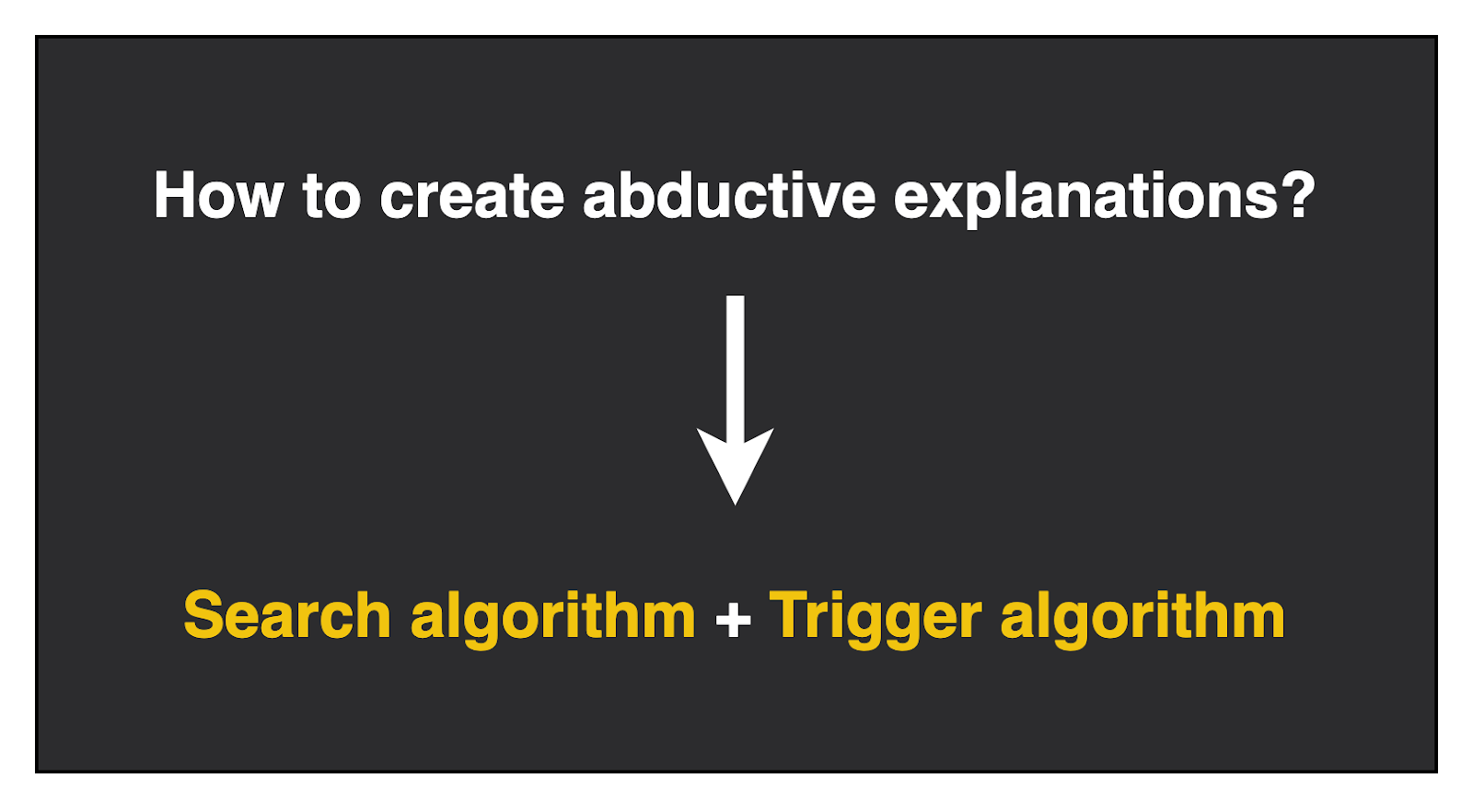# How to explain AI models?

Machine learning has become a cornerstone for product building with more and more companies have been investing and building AI models to improve their business outcomes. Building a model is one thing, but explaining it is just as important. There are many cases where explaining why your model has predicted an outcome can help the data scientist and the user to understand the reasoning behind a decision made by the AI model.Machine learning models can be like a black box. We do not know how the output is generated.

This is called Explainable AI. It is a new field of research which aims to find ways to explain AI using different methods. One way to explain AI models is by using Abductive Explanations.

## What are abductive explanations?

Abductive explanations use logic to explain why an event has occurred. For example, let us assume that you have built a model using the using dataset of passenger deaths and survivals from the Titanic and it predicts whether a passenger has survived the titanic. An example of an abductive explanation is as follows:

Given a passenger who is 18 years old, is a male and has 3 siblings, this passenger is predicted to have survived because he is male and is aged 18.Example abductive explanations. This can be written as a propositional formula.

There are 3 key notes about this explanation: it provides reasoning on why the model predicted a certain outcome in the form of a logic statement (i.e. is a male and is aged 18).
The explanation contains the same values as the given input (i.e. a passenger who is 18 years old, is a male and has 3 siblings).

The explanation must logically sound, meaning it must always be true for all inputs with the same input values (i.e. all passengers who are male and aged 18 survive the titanic).
Notice how the sibling feature has been dropped from the explanation. This is because even if whatever the value of ‘sibling’ is, the model will always trigger the same decision of ‘survive’, as long as the passenger is male and is 18 years old (this is known as being subset minimal).

These 3 points make up the definition of abductive explanations:

• The explanation contains the same values as the input
• The explanation is logically sound
• A minimal set of feature values is used

## Why use abductive explanations?One of the key benefits of an abductive explanation is the fact that you can trust its logical soundness. It is created in such a way that there are no counterexamples which have the same feature values and lead to a different outcome. Take an example from above, the explanation (is a male and is aged 18) will always lead to the passenger surviving based on the model. This allows the user to trust that the explanation will always lead to the same outcome.

## How to create abductive explanations?To generate abductive explanations, one needs to search for which variables belong to an explanation (Search algorithm) and check if these variables follow the definition of abductive explanations (Trigger algorithm). Given a set of feature input values in your model, you can iterate through each variable and answer the following question:
Is this variable part of my explanation?

If it is part of your explanation, then you can keep this feature and if not, then you can drop this feature. But what does it mean to have a variable that is part of your explanation? This is known as the trigger problem, which aims to check whether dropping the variable will still trigger the same decision.

Let’s take the previous titanic example. Assume that we have iterated through the gender variable and it is kept in my explanation (i.e. “is a male” is part of the explanation) and I am looking at the age feature (which is 18). If we were to drop the age feature, it is possible that my model will not trigger the same decision of ‘survived’ because there is an instance where age is not 18 and it leads to a decision of ‘not survived’. In order to keep the explanation logically sound, age must be kept in the explanation to trigger the same decision.

This iterative process of calling the solver for the trigger problem is all that is needed to create abductive explanations. However, creating an algorithm that checks if a counter-example exists can be worst-case exponential time (depending on the model type), since one needs to iterate through all the possible values in your feature space to search for a counter-example.

There are some constraints and clever tricks that can be placed to address this such as having a finite bound for features and using optimisation solvers like linear programming.

## Conclusion

Abductive explanation is a powerful way to explain AI models because you can guarantee their logical soundness. By using logic to explain your model, it is easy to understand for both technical and non-technical users.

---

AI: Why Does It Matter?: https://open.bulbapp.io/p/655eb80f-f558-455f-b8dc-d70af0b2f9c6/ai-why-does-it-matter
Ethics of Artificial Intelligence (AI): https://open.bulbapp.io/p/3d957673-f16e-45f9-8bfd-85ef0f884ea7/ethics-of-artificial-intelligence-ai
Python Machine Learning: https://open.bulbapp.io/p/41a73a45-7964-439d-b504-5acf9a5a10f7/python-machine-learning